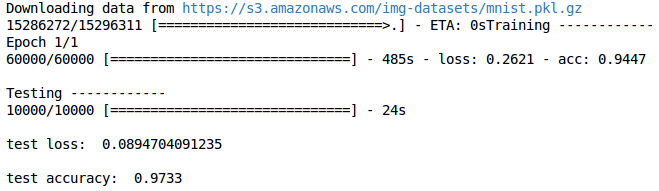# CNN 卷积神经网络

## 搭建模型 ¶

from keras.layers import Dense, Activation, Convolution2D, MaxPooling2D, Flatten


model.add(Convolution2D(
batch_input_shape=(64, 1, 28, 28),
filters=32,
kernel_size=5,
strides=1,
data_format='channels_first',
))


model.add(MaxPooling2D(
pool_size=2,
strides=2,
data_format='channels_first',
))


model.add(Convolution2D(64, 5, strides=1, padding='same', data_format='channels_first'))
model.add(MaxPooling2D(2, 2, 'same', data_format='channels_first'))


model.add(Flatten())


model.add(Dense(10))


model.compile(optimizer=adam,
loss='categorical_crossentropy',
metrics=['accuracy'])


## 训练 ¶

model.fit(X_train, y_train, epoch=1, batch_size=32,)